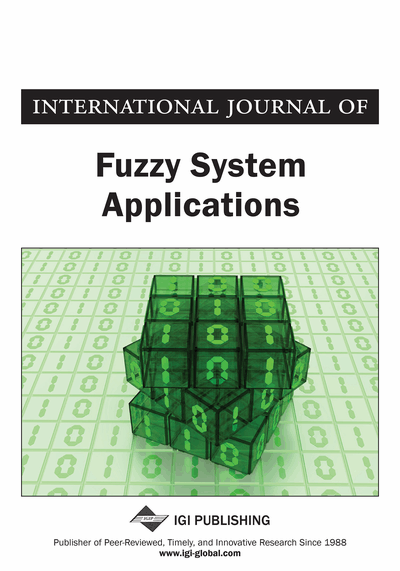# Using Fuzzy Goal Programming Technique to Solve Multiobjective Chance Constrained Programming Problems in a Fuzzy Environment

Animesh Biswas, Nilkanta Modak
DOI: 10.4018/ijfsa.2012010105
OnDemand:
(Individual Articles)
Available
\$37.50
No Current Special Offers

## Abstract

In this paper a fuzzy goal programming technique is presented to solve multiobjective decision making problems in a probabilistic decision making environment where the right sided parameters associated with the system constraints are exponentially distributed fuzzy random variables. In model formulation of the problem, the fuzzy chance constrained programming problem is converted into a fuzzy programming problem by using general chance constrained methodology. Then by realizing the fuzzy nature of the parameters associated with the system constraints, the problem is decomposed by considering the tolerance ranges of the parameters. The tolerance membership functions of each of the individual objectives are defined in isolation to measure the degree of achievements of the goal levels of the objectives. Then a fuzzy goal programming model is developed to achieve the highest degree of each of the defined membership functions to the extent possible. In the solution process the minsum fuzzy goal programming technique is used to find the most satisfactory decision in the decision making environment. An example is solved to illustrate the proposed methodology and the achieved solution is compared with the solution of another existing technique.
Article Preview
Top

## Introduction

To resolve infeasible liner programming problems, the concept of goal programming (GP) was first introduced by Charnes and Cooper (1961). Afterwards the remarkable methodological up gradation of GP was made by Ijiri (1965), Ignizio (1976) and other pioneer researchers. As a promising tool for solving problems having multiplicity of objectives with conflicting behaviors, GP has been studied extensively and widely circulated in the literature (Kornbluth & Steuer, 1981; Romero, 1986).

The main advantage of GP method is that it leads to arrive at an acceptable compromise solution directly. However the main weakness of classical GP is that the aspiration levels of the goals need to be specified precisely in making a decision. Also the classical GP technique cannot capture directly the uncertainty arises due to the presence of chance constraints associated with the multiobjective decision making problems. To overcome such uncertainties due to probabilistic nature, Charnes and Cooper (1959) first introduced chance constrained programming (CCP) technique. Thereafter different methodological aspects of CCP was discussed by Kataoka (1963), Miller and Wagner (1965), Panne and Popp (1963), Prékopa (1973) and other researchers. Contini (1978) developed an algorithm for stochastic GP problems when the random variables are normally distributed with known means and variances. Stancu-Minasian and Wets (1976) and Maarten and Van Der (2003) discussed research bibliographical surveys in the field of stochastic programming.

Stochastic programming deals with the theory and methods of incorporating stochastic variables into a mathematical programming problem. In most of the stochastic programming approaches, the basic concept is to convert the probabilistic nature of the parameters into an equivalent deterministic model according to the specified confidence levels and the nature of the distribution followed by the random variables.

In practical decision making situation, whether it is probabilistic or deterministic or both, other kind of uncertainty arises due to decision maker’s (DM’s) imprecise understanding of the nature of parameters involved with the problem which are very often ambiguously defined. To overcome the above difficulty the concept of fuzzy set theory, initially proposed by Zadeh (1965), has been introduced in the field of chance constrained multiobjective decision making environment where aspiration level of the goal of the objectives and the aspiration level of parameters involved with the chance constraints are assigned in an imprecise manner. As a result the methodologies developed only for solving stochastic programming problem cannot perform well and also fail to provide satisfactory answers.

To overcome such a situation, Ludhandjula (1983) introduced a fuzzy stochastic programming methodology. Chakravorty (2002) studied CCP in fuzzy environment by considering aspiration level and utility function for objective function and fuzzy probability involved with the system constraints. Sinha et al. (1998) presented a methodology for solving multiobjective chance constrained fuzzy linear programming.

## Complete Article List

Search this Journal:
Reset
Volume 12: 1 Issue (2023)
Volume 11: 4 Issues (2022)
Volume 10: 4 Issues (2021)
Volume 9: 4 Issues (2020)
Volume 8: 4 Issues (2019)
Volume 7: 4 Issues (2018)
Volume 6: 4 Issues (2017)
Volume 5: 4 Issues (2016)
Volume 4: 4 Issues (2015)
Volume 3: 4 Issues (2013)
Volume 2: 4 Issues (2012)
Volume 1: 4 Issues (2011)
View Complete Journal Contents Listing